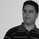한국어
TradingView
12205 뷰
12205
This is my second Version of Heikin Ashi Strategy.

Changes:
-Different Time Frame for Fast EMA and Slow EMA
-MACD Filter with own Time Frame

You can tweak around with settings , but defaults gives good results in Forex,Stocks and Index.

I also made an Alert Script
```//@version=2
//Heikin Ashi Strategy  V2 by breizh29

strategy("Heikin Ashi Strategy  V2",shorttitle="HAS V2",overlay=true,default_qty_value=1000,initial_capital=100000,currency=currency.EUR)
res = input(title="Heikin Ashi Candle Time Frame", type=resolution, defval="60")
hshift = input(1,title="Heikin Ashi Candle Time Frame Shift")
res1 = input(title="Heikin Ashi EMA Time Frame", type=resolution, defval="180")
mhshift = input(0,title="Heikin Ashi EMA Time Frame Shift")
fama = input(1,"Heikin Ashi EMA Period")
test = input(1,"Heikin Ashi EMA Shift")
sloma = input(30,"Slow EMA Period")
slomas = input(1,"Slow EMA Shift")
macdf = input(false,title="With MACD filter")
res2 = input(title="MACD Time Frame", type=resolution, defval="15")
macds = input(1,title="MACD Shift")

//Heikin Ashi Open/Close Price
ha_t = heikinashi(tickerid)
ha_open = security(ha_t, res, open[hshift])
ha_close = security(ha_t, res, close[hshift])
mha_close = security(ha_t, res1, close[mhshift])

//macd
[macdLine, signalLine, histLine] = macd(close, 12, 26, 9)
macdl = security(ha_t,res2,macdLine[macds])
macdsl= security(ha_t,res2,signalLine[macds])

//Moving Average
fma = ema(mha_close[test],fama)
sma = ema(ha_close[slomas],sloma)
plot(fma,title="MA",color=lime,linewidth=2,style=line)
plot(sma,title="SMA",color=red,linewidth=2,style=line)

//Strategy
golong =  crossover(fma,sma) and (macdl > macdsl or macdf == false )
goshort =   crossunder(fma,sma) and (macdl < macdsl or macdf == false )

strategy.entry("Buy",strategy.long,when = golong)
strategy.entry("Sell",strategy.short,when = goshort)

```Hello,
may you please precise how the "ongoing" fma is calculated?
Let's assume the n-1 period of 180 minutes is ended. We are entering in the new period of 180, which consists of 12 periods of 15 minutes. Plotting the fma on 15 minutes timeframe, I do see it is calculated "ongoing", but I do not understand what "close" and "previus EMA" values it is considering (EMA formula = * (2 / n+1) + previous EMA.)
Thanks,
Aldo
응답There is a bug in this strategy. This strategy is trading by looking at the future closing price.
Example : 24 Mar 16' 22:15, fma is 1.1169. But, fma has a higher value when calculated from open, high, low, close in the past 3 hours.
(this calculation of this strategy includes 25 Mar 16' 00:45)

But you can still get profits.
응답Hi, very good trading system. Have you this trading system to MT5? Thank's.
응답
KO 한국어
EN English
EN English (UK)
EN English (IN)
DE Deutsch
FR Français
ES Español
IT Italiano
PL Polski
SV Svenska
TR Türkçe
RU Русский
PT Português
ID Bahasa Indonesia
MS Bahasa Melayu
TH ภาษาไทย
VI Tiếng Việt
JA 日本語
ZH 简体中文
ZH 繁體中文
AR العربية
HE עברית